# Student Report – Reading Progress Indicator

Use this reports to review gains and detailed assessment results for an individual student, as measured by Reading Progress Indicator (RPI), our integrated assessment tool. RPI assessments help measure the impact of Fast ForWord program intervention. To learn about the assessments in this report see About Reading Progress Indicator (RPI).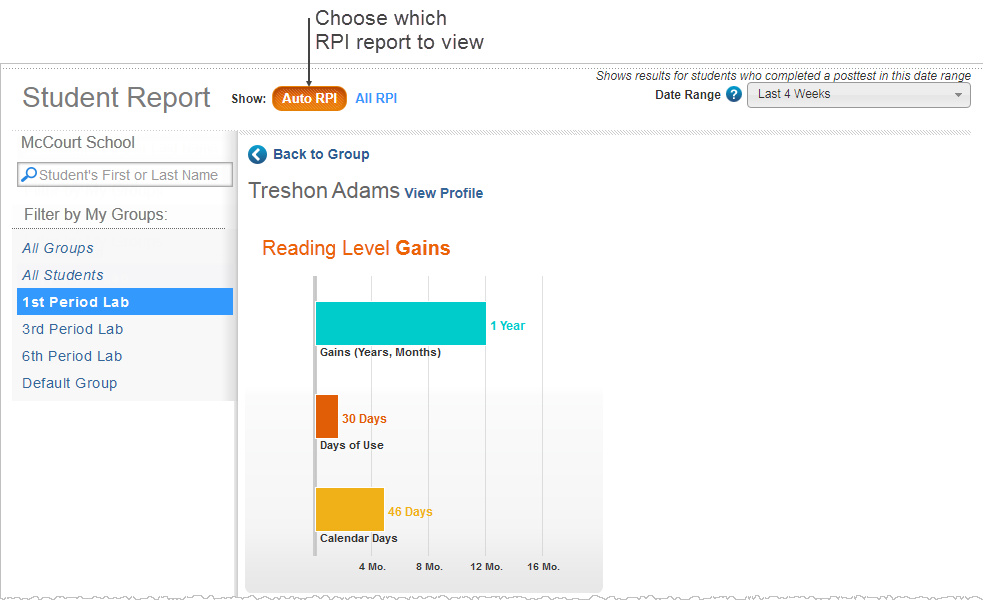This bar graph illustrates the student's reading level gain score compared to the length of time spent using the Fast ForWord programs, as measured between the student's initial assessment and latest follow-up assessment completed within the date range.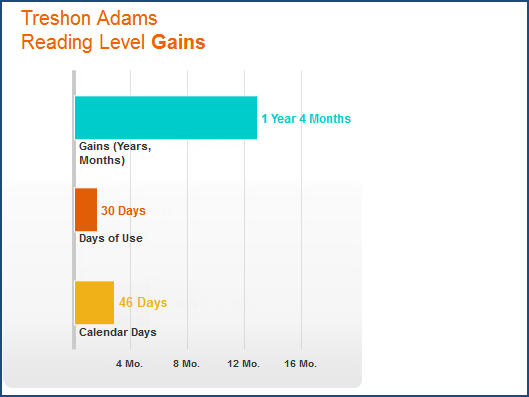Gains. This bar represents the reading level gain score after Fast ForWord program use. mySciLEARN calculates this score by subtracting the student's reading level on their initial assessment from the reading level on the student's latest follow-up assessment. A numerical gain score appears at the end of the bar, in years and months. To learn more see Gain scores.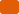Days of Use. This bar represents the number of days the student worked on the Fast ForWord programs, as measured between the assessments. This number is generally lower than the number of calendar days due to weekends, holidays, and absences. The actual number of participation days appears at the end of the bar.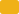Calendar Days. This bar represents the number of calendar days that have elapsed since the student first started the Fast ForWord programs, as measured between the assessments. The actual number of calendar days appears at the end of the bar.

In the gains reports, reading level gain scores are the same as grade equivalent gain scores.

## Product Usage table

This table breaks down the number of training days for each Scientific Learning program used by the student, as measured between the student's assessments.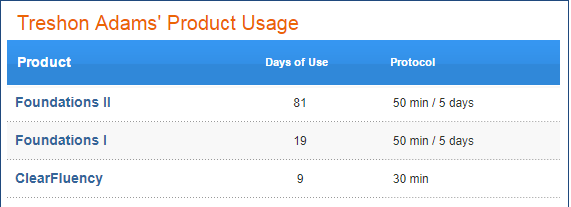Product. The Scientific Learning programs used by the student. Click the column header to sort the report by program name. Click again to reverse the sort order.

Days of Use. The actual number of days that the student used each program, as measured between the assessments.

Protocol. The protocol (or schedule) that the student used when working on each of the Scientific Learning programs. If the student used more than one protocol with a program, this column lists those protocols, with the most recent protocol listed first (includes the last three protocols only). Protocols provide specific training schedules for the student. To learn more see Fast ForWord program protocols and Reading Assistant Plus Schedules.

## Reading Progress Indicator Test History table

This table presents the results for each RPI assessment taken by the student, providing an in-depth look at the student's growth as he or she progresses through the Fast ForWord programs. The first row in the table presents the student's initial assessment results. Each subsequent row presents the student's follow-up assessment results (up to the latest follow-up assessment completed within the date range), along with gain scores that show how much the student has improved since the previous assessment. This provides an historical record of the student's growth between assessments and across Fast ForWord program use.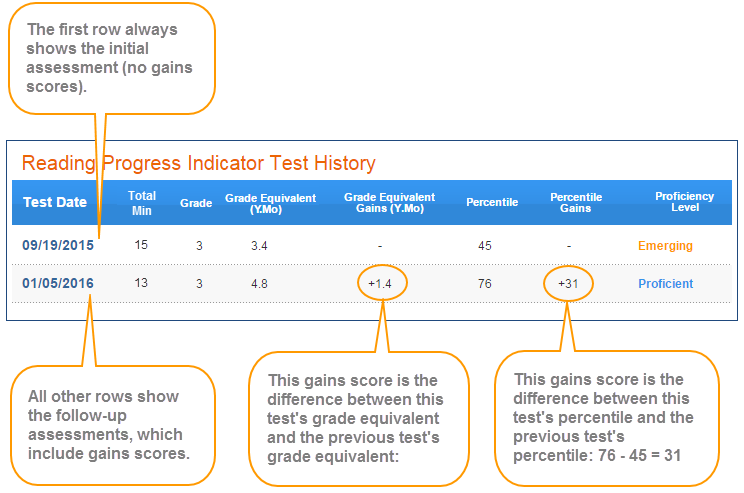The table includes the following columns. To sort the table, click the Test Date column header.

Test Date. The date that the student completed each assessment included in the table.

Total Min. Time spent taking the assessment, rounded to the nearest minute.

Grade Equivalent (Y.Mo). The student's RPI grade equivalent for the assessment. This score reflects the student's performance compared to the average student at a given grade level. The first digit in this score represents the year and the second digit represents the month. The grade equivalent score is based on an academic school year of ten months, so a student with a score of 2.4 is performing on par with an average student in the fourth month of the second grade. To learn more see Grade equivalent scores.

Grade Equivalent Gains (Y.Mo). The student's RPI grade equivalent gain for each follow-up assessment included in the table, as measured between the previous assessment and that follow-up assessment. Grade equivalent scores reflect the student's performance compared to the average student at a given grade level. This gain score shows the difference between the student's initial assessment's score and that test's score. The first digit in this score represents the number of years gained and the second digit represents the number of months gained. The grade equivalent score is based on an academic school year of ten months, so a score of 2.4 represents a gain of two academic years and four months. To learn more see Gain scores.

Percentile Gains. The student's reading level percentile gain for each follow-up assessment, as measured between the previous assessment and that follow-up assessment.

Proficiency Level - The student's reading proficiency level for each assessment. Proficiency levels are based on percentile scores and can reflect how each student compares with his or her peers across the nation. Note that students can make dramatic gains without changing performance levels; for example, a student could move from the 55th percentile to the 75th percentile but still be within the Proficient level. To learn more see Reading proficiency levels.

• Struggling (red) – 1st to 29th percentile
• Emerging (yellow) – 30th to 54th percentile
• Proficient (blue) – 55th to 79th percentile
• Advanced (green) – 80th to 99th percentile

## Performance by Reading Skill table

The Performance by Reading Skill table presents reading skill results for each RPI assessment taken by the student.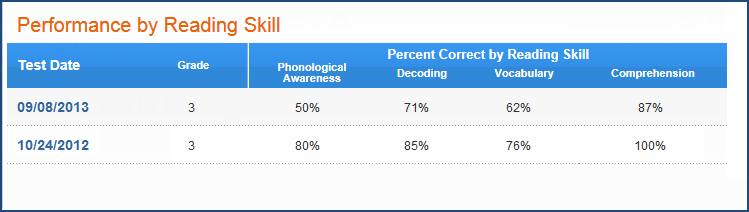The table includes the following columns. Each row corresponds to the same row in the Reading Progress Indicator Test History table.

Test Date. The date that the student completed the assessment.